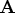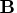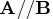Previous Page | Next Page

 Language Reference

## Concatenation Operator, Vertical:   //

concatenates matrices vertically

matrix1//matrix2

The vertical concatenation operator (//) produces a new matrix by vertically joining matrix1 and matrix2. Matrix1 and matrix2 must have the same number of columns, which is also the number of columns in the new matrix. For example, ifhas three rows and two columns andhas four rows and two columns, thenproduces a matrix with seven rows and two columns. Rows 1 through 3 of the new matrix correspond to; rows 4 through 7 correspond to.

For example, the following statements produce the matrix, as shown:

```
a={1 1 1,
7 7 7};
b={0 0 0,
8 8 8};
c=a//b;
```

```
C             4 rows      3 cols    (numeric)

1         1         1
7         7         7
0         0         0
8         8         8
```
Now, let
```
b={"AB" "CD",
"EF" "GH"};
c={"I" "J",
"K" "L",
"M" "N"};
```
In this case, the following statement produces the matrix, as shown:
```
a=b//c;
```

```
A        5 rows      2 cols    (character, size 2)

AB CD
EF GH
I  J
K  L
M  N
```

For character matrices, the element size of the result matrix is the larger of the element sizes of the two operands.

You can use the vertical concatenation operator when one of the arguments has not been assigned a value. For example, ifhas not been defined andis a matrix,//results in a new matrix equal to.

Quotation marks (") are needed around matrix elements only if you want to embed blanks or maintain uppercase and lowercase distinctions.Previous Page | Next Page | Top of Page